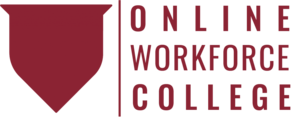Introduction to Basic Math JC-MTH-1001 is a Course

Introduction to Basic Math JC-MTH-1001

Self-paced

Full course description

Course Description

Good math skills are essential for your success in manufacturing. This course introduces resources you will need when working with math.

By the end of this course, you will be able to

• Identify the different branches of mathematics

• Use the calculator

• Look up terms in the glossary

• Understand the importance of estimating an answer before calculating it

Estimated completion time (hours): 0.7